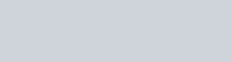Draw the graphs of the equations 5x − y = 5 and 3x − y = 3.
Question:

Draw the graphs of the equations 5x − y = 5 and 3x − y = 3. Determine the co-ordinates of the vertices of the triangle formed by these lines and y-axis. Calculate the area of the triangle so formed.

Solution:

The given equations are:

$5 x-y=5$$. .(i)$

$3 x-y=3$….(ii)

Putting $x=0$ in equation (i) we get:

$\Rightarrow 5 \times 0-y=5$

$\Rightarrow y=-5$

$x=0, \quad y=-5$

Putting $y=0$ in equation (i) we get:

$\Rightarrow 5 x-0=5$

$\Rightarrow x=1$

$x=1, \quad y=0$

Use the following table to draw the graph.Draw the graph by plotting the two points $A(0,-5), B(1,0)$ from table$3 x-y=3$

Putting $x=0$ in equation $(i i)$ we get:

$\Rightarrow 3 \times 0-y=3$

$\Rightarrow y=-3$

$x=0, \quad y=-3$

Putting $y=0$ in equation $(i i)$, we get:

$\Rightarrow 3 x-0=3$

$\Rightarrow x=1$

$x=1, \quad y=0$

Use the following table to draw the graph.Draw the graph by plotting the two points $C(0,-3), D(1,0)$ from table.

Hence the vertices of the required triangle are $B(1,0), C(0,-3)$ and $A(0,-5)$.

Now,

$\Rightarrow$ Required area $=$ Area of $\mathrm{PCA}$

$\Rightarrow$ Required area $=1 / 2($ base $\times$ height $)$

$\Rightarrow$ Required area $=1 / 2(2 \times 1)$ sq. units

Hence the required area is 1 sq.units